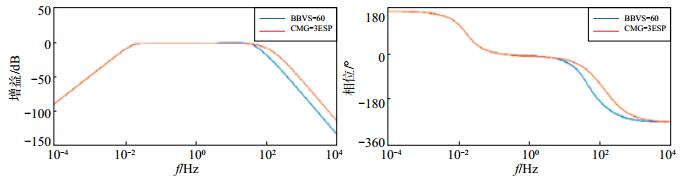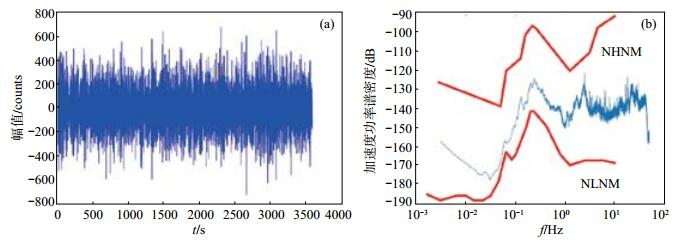﻿ 数字地震仪频响特性与地动噪声功率谱计算文章快速检索
 地震地磁观测与研究2018, Vol. 39Issue (1): 96-101  DOI: 10.3969/j.issn.1003-3246.2018.01.0160

### 引用本文Li Xingquan, Wu Peng, Shao Yuping, et al. Calculation of frequency characteristics of digital seismograph and the power spectra of ground motion noises based on Matlab[J]. Seismological and Geomagnetic Observation and Research, 2018, 39(1): 96-101. DOI: 10.3969/j.issn.1003-3246.2018.01.016.

### 文章历史

Calculation of frequency characteristics of digital seismograph and the power spectra of ground motion noises based on Matlab
Li Xingquan, Wu Peng, Shao Yuping, Tang Lin
Sichuan Earthquake Agency, Chengdu 610041, China
Abstract: Seismograph system is a linear stable dynamic system, which can be described by the amplitude-frequency and phase-frequency characteristics. Based on the practical application, characteristics of seismograph system and transfer function of BBVS-60 and CMG-3ESP are analyzed in this paper, and the mathematical explanation in the process of data processing is introduced. With amazing computing capacity of Matlab, frequency characteristic curve of digital seismograph is drawn and the power spectra values of ground motion noises are calculated. The method also provides a reference for further work such as analysis of the frequency response characteristics of seismometer and the noise level test of seismic station survey.
Key Words: Bode function    frequency response    noise power spectrum    digital seismograph
0 引言

1 频域分析原理 1.1 地震仪频率响应

 $\begin{array}{l} 幅频特性\;\;\;\;\;\;\;\;\;\;\;\;\;\;\;\;\;\;A\left(\omega \right) = {A_2}\left(\omega \right)/{A_1}\\ 相频特性\;\;\;\;\;\;\;\;\;\;\;\;\;\;\;\;\;\;\varphi \left(\omega \right) = {\varphi _2}\left(\omega \right) - {\varphi _1} \end{array}$ (1)

 $\begin{array}{l} G\left({j\omega } \right) = A\left(\omega \right){e^{j\varphi }}\left(\omega \right)\\ G\left({j\omega } \right) = A\left(\omega \right)\angle \varphi \left(\omega \right) \end{array}$ (2)

1.2 地震台站功率谱密度函数

 $\hat P\left({{e^{j\omega }}} \right) = \frac{1}{N}{\left| {{X_N}\left({{e^{j\omega }}} \right)} \right|^2}$ (3)

2 利用Matlab进行频域分析 2.1 Bode图和Bode函数

Bode图又称对数频率特性图，由2部分组成：①对数幅频特性曲线；②对数相频特性曲线。Bode图横坐标通常以ω为横轴变量，并采用ω的对数值刻度进行标注，变化范围较广（施梨，2015）。幅值和相位的Bode图横坐标均采用ω表示，纵坐标分别采用L(ω) = 20lg|G()|或φ(ω) = ∠G ()表示。

Matlab提供的Bode函数可以直接求取、绘制给定线性系统的Bode图。当Bode命令不包含左端返回变量时，函数运行后在屏幕上直接绘制Bode图。如命令表达式的左端含有返回变量，Bode函数计算出的幅值和相角将返回相应矩阵，屏幕上不显示频率响应图，因此可通过相应的调用命令，取出对应幅值或相角信息进行计算，命令的调用格式为

[mag, phase, w] = bode(num, den)

[mag, phase, w] = bode(num, den, w)      或[mag, phase, w] = bode(G)

[mag, phase, w] = bode(G, w)

2.2 地震计幅频特性和地动噪声功率谱计算

BBVS-60宽频带地震计和CMG-3ESP宽频带地震计具有频带宽、灵敏度高、动态范围大的特点，传递函数稳定。目前，2种类型地震计在中国区域地震监测台网使用广泛。根据厂家给定的地震仪传递函数和零极点，借助Matlab，可获得设备的频响曲线，进而直观对比设备是否符合工作要求或多种设备的频响差异。

CMG-3ESP和BBVS-60地震计的零极点和归一化因子（戴伳贵，2009）见表 1表 1 CMG -3ESP、BBVS -60地震计零极点和归一化因子 Tab.1 Zeros poles and normalization factor of CMG-3ESP and BBVS-60 seismograph图 1 BBVS-60和CMG-3ESP地震计频响曲线 Fig.1 Frequency response curve of the BBVS-60 and CMG-3ESP seismograph

[num1, den1]=zp2tf(z1, p1, k1);

sys1=tf(num1, den1);

bode(sys1);

 $地动速度\;\;\;\;\;\;\;\;\;\;\;\;\;\;\;\;\;\;{X_v} = {A_{v0}} \times {K_{{\rm{ad}}}}/\left({G \times {S_{{\rm{s0}}}}} \right)$ (4)
 $地动加速度\;\;\;\;\;\;\;\;\;\;\;\;\;\;\;\;\;{X_a} = \omega \times {A_{v0}} \times {K_{{\rm{ad}}}}/\left({G \times {S_{{\rm{s0}}}}} \right)$ (5)

 $P\left({{e^{j\omega }}} \right) = \hat P\left({{e^{j\omega }}} \right)/{\left| {G\left({j\omega } \right)} \right|^2}$ (6)图 2 垂直向原始信号波形及噪声功率谱曲线 (a)原始信号波形；(b)噪声功率谱 Fig.2 Original signal waveform and power spectra of noise of vertical component

z=[0;0];

p=[-7.40159e-02+7.40159e-02i;-7.40159e-02-7.40159e-02i;...

-1.0053088e+03; -5.026544e+02;...

-1.1309274e+03];

k=+5.76e+8;

[num, den]=zp2tf(z, p, k);

sys1=tf(num, den);

[mag phase]=bode(sys1, 2*pi*f);

mag1(1:nfft/2+1)=mag(1, 1, 1:nfft/2+1);

mag1=mag1.*mag1;

pxx2=pxx2./abs(mag1');

3 结束语

 陈祥熊, 陈文明, 江燕, 等. 福建数字地震台网台址背景噪声分析[J]. 地震地磁观测与研究, 1998, 19(5): 13-22. 戴伳贵, 谌亮, 康宏, 等. CMG-3ESPC宽频带地震计稳态标定和传递函数构建[J]. 四川地震, 2009, 11061106(3): 12-15. 胡寿松. 自动控制原理[M]. 北京: 国防工业出版社, 1994. 邵玉平, 韩进, 宋澄. 向家坝数字遥测地震台网的台基地噪声分析[J]. 地震地磁观测与研究, 2007, 28(2): 61-64. 施梨. Matlab工程仿真与应用30例[M]. 北京: 电子工业出版社, 2015. 徐嘉隽. 福建测震台网测震台站环境地噪声水平[J]. 地震地磁观测与研究, 2013, 34(1/2): 101-105. 张志涌. 精通Matlab R2011a[M]. 北京航空航天大学出版社, 2011. 中国地震局监测预报司. 数字地震观测技术[M]. 北京: 地震出版社, 2003. Wunsch D C, Bell R R. Determination of threshold failure levels of semiconductor diodes and transistors due to pulse voltages[J]. IEEE Transactions on Nuclear Science, 1968, 15(6): 244-259. DOI:10.1109/TNS.1968.4325054